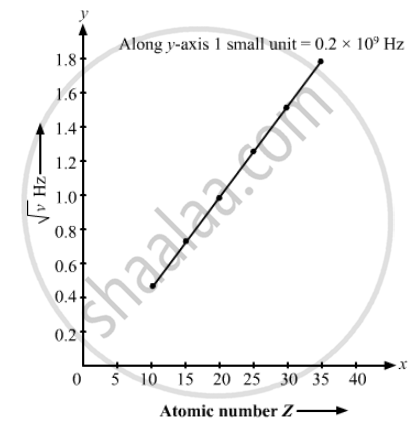Department of Pre-University Education, KarnatakaPUC Karnataka Science Class 12
Share

# The Kβ X-rays from Certain Elements Are Given Below. Draw a Moseley-type Plot of √V Versus Z for Kβ Radiation. - Physics

ConceptElectromagnetic Spectrum

#### Question

The Kβ X-rays from certain elements are given below. Draw a Moseley-type plot of √v versus Z for Kβ radiation.

 Element Ne P Ca Mn Zn Br Energy (keV) 0.858 2.14 4.02 6.51 9.57 13.3

#### Solution

We can directly get value of v by energy frequency relation.
hv = energy
Here, h = Planck's constant

v = "Energy (in  keV)"/h

The required graph is as follows :Is there an error in this question or solution?

#### Video TutorialsVIEW ALL 

Solution The Kβ X-rays from Certain Elements Are Given Below. Draw a Moseley-type Plot of √V Versus Z for Kβ Radiation. Concept: Electromagnetic Spectrum.
S